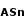# Number Arcsine node

Left Toolbar >Math Nodes >Number Arcsine

The Number Arcsine node allows you to calculate the arcsine of a number.

## Procedure

### To calculate a Number Arcsine in the node graph:

1. On the left toolbar choose Math Nodes> Number Arcsine2. Drag the Number Arcsine icon into the Node Graph.3. Connect the Output: Result.

## Inputs

Number

A NUMBER type of value.

## Outputs

Result

The number value.

## Attributes PanelNumber

Number value. This attribute is exposed as input by default.

Math Nodes

Main Page